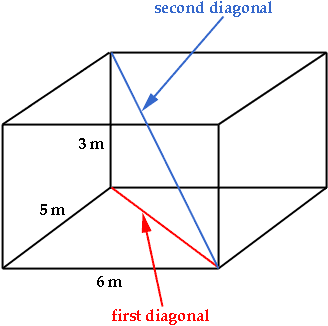Quandaries and Queries Hiya! Name's Miki and I'm currently in grade 9. I've got this question I was supposed to do for homework but math's just not my strong point. The question is simply and I the way to go about it simpler: A room is 6m long, 5m wide and 3 m high. Find the distance from the corner of the floor to the opposite corner of the celing. PS I REALLY REALLY need to know hot to figure out the answer for this in 9 hours time!!! (don't know what time zone it is there) By then if no one has answered this, don't bother but thanks anyway. Hi miki, You need to use the theorem of Pythagoras twice to solve this problem. Imagine that you are in the room. Take red marker and draw a line on the floor from one corner to the opposite corner. In the diagram below I called this the first diagonal. You now have a right triangle with legs of length 5 m and 6 m, and hypotenuse the first diagonal. Use the theorem of pythagoras to find the length of the hypotenuse.Look at the diagram again. There is a second right triangle. Its legs are a line of length 3 m, and the first diagonal. The hypotenuse, which I drew in blue, is a line from a corner of the room to the opposite corner of the ceiling. Since you know the lengths of the legs you can use Pythagoras' Theorem to find the length of the second diagonal. Cheers, Penny Go to Math Central